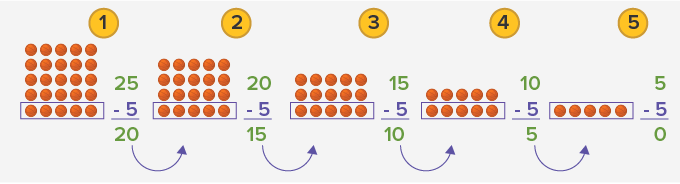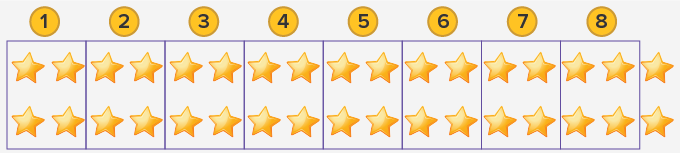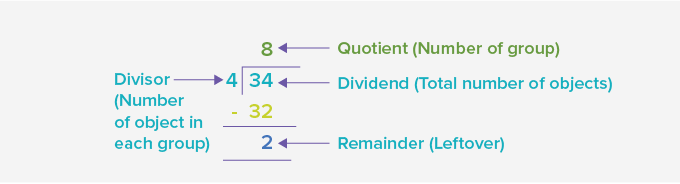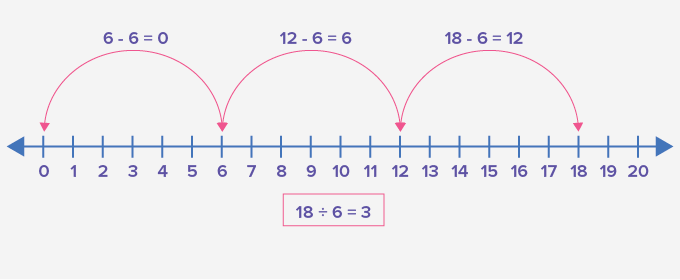# Repeated Subtraction - Definition with Examples

The Complete K-5 Math Learning Program Built for Your Child

• 40 Million Kids

Loved by kids and parent worldwide

• 50,000 Schools

Trusted by teachers across schools

• Comprehensive Curriculum

Aligned to Common Core

## What is Repeated Subtraction?

Repeated subtraction is a method of subtracting the equal number of items from a larger group. It is also known as division.

If the same number is repeatedly subtracted from another larger number until the remainder is zero or a number smaller than the number being subtracted, we can write that in the form of division.

For example:

If there are 25 balls and we form a group of 5 balls each.Here, the number 5 has been repeatedly subtracted 5 times. We can say that the number 5 has been subtracted 5 times from 25. So, we can write this subtraction as 25 ÷ 5 = 5.

Similarly, to solve a division problem through repeated subtraction, we repetitively group and subtract the same number again and again to find the answer.

Here are a few examples of repeated subtraction.

There are 34 stars. How many groups of 4 stars in each can be formed?In the given image we can see 34 stars. Now, using repeated subtraction, we can group them in smaller groups of 4 stars in each group. We can start to subtract 4 stars repeatedly until we are left with 0 or a number less than 4.34 - 4 = 30     30 - 4 = 26   26 - 4 = 22    22 - 4 = 18  18 - 4 = 14   14 - 4 = 10  10 - 4 = 6    6 - 4 = 2

We get 8 groups of 4 and with 2 stars remaining.

This example can be mathematically written as 34 ÷ 4. Where 34 is the dividend. The divisor is the number of stars in each group, that is, 4. The number of times 4 is subtracted is the quotient. So, 8 is the quotient and the leftover stars are the remainder. So, 2 is the remainder.Since repeated subtraction is division, it can be written in 2 ways.

Example: Let’s say there are 18 items. These can be written in 2 ways as shown.

 18 ÷ 6 18 ÷ 3When the divisor is 6, we make groups of 6. We get, 3 groups of 6. So, 18 ÷ 6 = 3 When the divisor is 3, we make groups of 3. We get, 6 groups of 3. So, 18 ÷ 3 = 6

 Fun fact: Repeated subtraction is like jumping back from the bigger number until you get a zero or a smaller number.18 – 6 = 12       →          12 – 6 = 6         →      6 – 6 = 0

or

18 ÷ 6 = 3

Won Numerous Awards & Honors